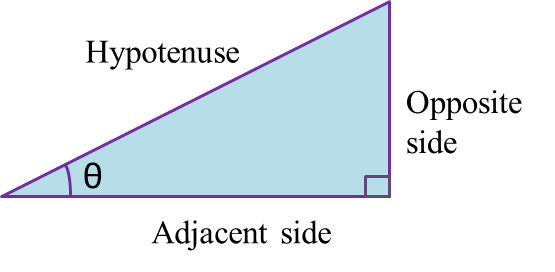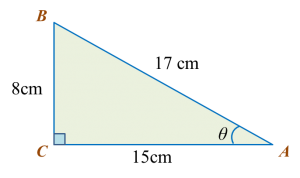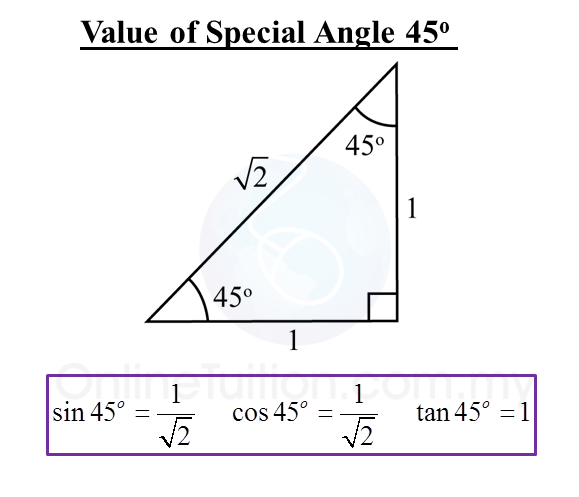15.1 Trigonometry

15.1 Trigonometry

15.1.1 Trigonometrical Ratios of an Acute Angle

1.2.   Hypotenuse is the longest side of the right-angled triangle which is opposite the right angle.
3.   Adjacent side is the side, other than the hypotenuse, which has direct contact with the given angle, θ.
4.   Opposite side is the side which is opposite the given angle, θ.
5.   In a right-angled triangle,

6.
When the size of an angle θ increases from 0o to 90o,

· sin θ increases.
· cos θ increases.
· tan θ increases.

7.   The values of sin θ, cos θ and tan θ remain the same even though the size of the triangle has changed.
Example:Find the sine, cosine and tangent of the give angle, θ, in triangle ABC.

Solution:
$\begin{array}{l}\mathrm{sin}\theta =\frac{BC}{AB}=\frac{8}{17}\\ \mathrm{cos}\theta =\frac{AC}{AB}=\frac{15}{17}\\ tan\theta =\frac{BC}{AC}=\frac{8}{15}\end{array}$

15.1.2  Values of Tangent, Sine and Cosine
1.   The values of the trigonometric ratios of 30o, 45o and 60o (special angle) are as below.2.   1 degree is equal to 60 minutes.
1o= 60’

3.   A scientific calculator can be used to find the value of the sine, cosine or tangent of an angle.
Example:
sin 40.6o = 0.5954

 Calculator Computation     Press [sin] [40.6] [=] 0.595383839
4.   Given the values of sine, cosine and tangent, we can find the angles using a scientific calculator.
Example:
tan x = 1.7862
x = 67o30’

 Calculator Computation Press [shift] [tan][1.7862]  [=][o’’’]  67o30’